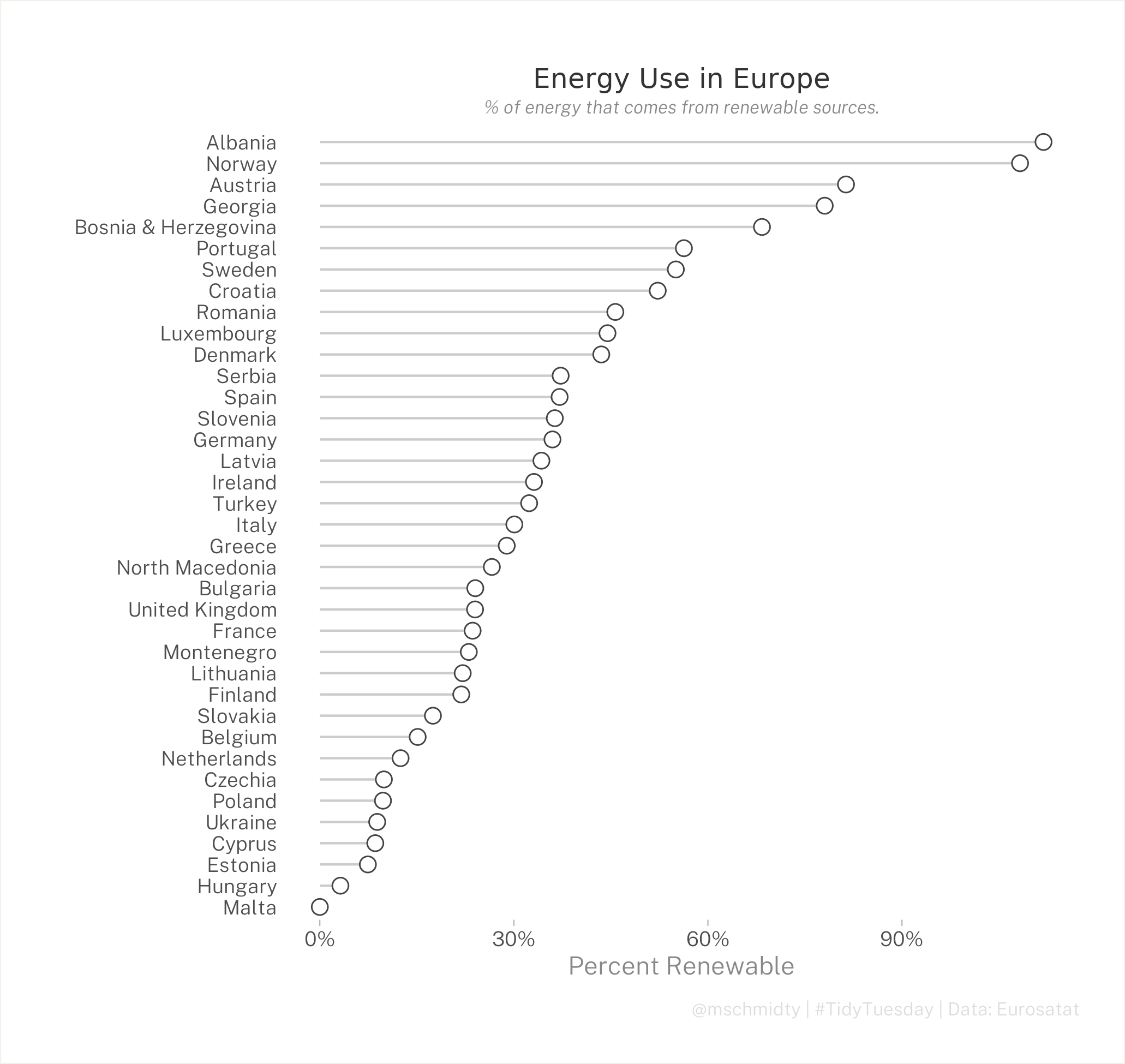I haven't had a ton of time lately. On a recent road trip, I tried out a Tidy Tuesday submission on European evergy usage. Because I didn't have enough time, I wanted to make something simple and work on making it really easy to read. I think I did that. I would have liked to do a bit more with the theme. Maybe next time.

I was inspired by @MaiaPelletier and her circular version using the same data - which is amazing.

The final product:And the scripts:

``library(tidytuesdayR)library(tidyverse)library(here)extrafont::loadfonts()theme_set(theme_light()+            theme(              text=element_text(family="Public Sans Light")            ))energy_data <- tidytuesdayR::tt_load('2020-08-04')energy_types <- energy_data\$energy_typescountry_totals <- energy_data\$country_totalscountry_totals%>%  count(level)total_energy<-country_totals%>%  select(-`2017`, -`2016`, -level)%>%  pivot_wider(names_from=type, values_from=`2018`)%>%  janitor::clean_names()%>%  mutate(total_energy_use = total_net_production+imports-exports-energy_absorbed_by_pumping)%>%  select(country, total_energy_use)percent_renewable<-energy_types%>%  select(-`2016`, -`2017`, -`level`)%>%  pivot_wider(names_from=type, values_from=`2018`)%>%  janitor::clean_names()%>%  mutate(    renewable=hydro+wind+solar+geothermal+pumped_hydro_power,     non_renewable = conventional_thermal+nuclear+other,    percent_renewable = renewable/(renewable+non_renewable)    )%>%  left_join(total_energy)%>%  mutate(    country_name=ifelse(is.na(country_name), "United Kingdom", country_name),    other_percent_renewable = renewable/total_energy_use)View(percent_renewable)percent_renewable%>%  mutate(country_name=fct_reorder(country_name, other_percent_renewable))%>%  ggplot(aes(country_name, other_percent_renewable))+  geom_segment( aes(x=country_name, xend=country_name, y=0, yend=other_percent_renewable), color="#CCCCCC") +  geom_point( color="#444444", size=3, shape=21, fill="#ffffff") +  coord_flip()+  theme(    plot.margin = margin(20, 20, 20, 20),    plot.background = element_rect(color="#F2F0EA"),    panel.background = element_blank(),    panel.grid.major.x = element_blank(),    panel.grid.major.y = element_blank(),    panel.grid.minor.x = element_blank(),    panel.border = element_blank(),    axis.line=element_blank(),    axis.ticks.y = element_blank(),    axis.text.x = element_text(size = 9),    axis.text.y = element_text(margin = margin(t = 30)),    axis.title = element_text(size=11, color="#888888"),    plot.title = element_text(hjust = 0.5,                               size = 12,                              family="Public Sans Bold",                              color="#333333",                              margin = margin(10, 0, 3, 0)),    plot.subtitle = element_text(hjust = 0.5,                                 size = 8,                                color = "#888888",                                  face = "italic"),    plot.caption = element_text(size=8,                                color = "#dddddd",                                margin = margin(10, 0, 0, 0))  )+  xlab("") +  ylab("Percent Renewable")+  labs(title="Energy Use in Europe",       subtitle="% of energy that comes from renewable sources.",       caption = "@mschmidty | #TidyTuesday | Data: Eurosatat")+ scale_y_continuous(labels = scales::percent)+  ggsave(here("Dropbox", "r", "tidy_tuesday", "figures", "europe_energy_v1.png"), type='cairo', height=6.5)``